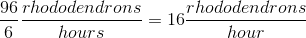## Example Questions

### Example Question #184 : Fractions

Simplify the following: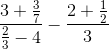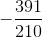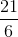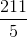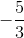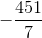Explanation:

This problem merely requires careful working out of each part. Begin by simplifying the first fraction: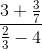The numerator will be: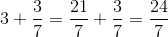The denominator will be: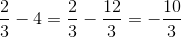Thus, we have the following fraction: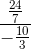Remember that you must multiply the numerator by the reciprocal of the denominator: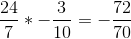Now, work on the second fraction: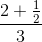This fraction is much easier. After simplifying the numerator, you get: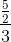This is the same as: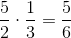Thus, we come to our original expression! It is: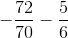The common denominator of these fractions is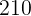. Thus, you have: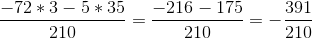### Example Question #601 : Arithmetic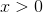Quantity A: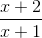Quantity B: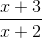Quantity A is greater.

Quantity B is greater.

The relationship between A and B cannot be determined.

The two quantities are equal.

Quantity A is greater.

Explanation:

As it is, quantities A and B have different denominators, so making a comparison can be tricky. Making a common denominator will allow for comparison of just the numerators, so that would make a good first step:

Quantity A: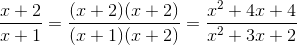Quantity B: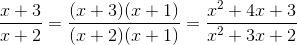Disregarding equal denominators, since they'll always have a positive value, compare the numerators. If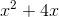is subtracted from quantity A and from quantity B, we're left withfor the former andfor the latter.

Quantity A is greater.

### Example Question #602 : Arithmetic

Quantity A: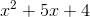Quantity B: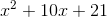Quantity A is greater.

Quantity A and Quantity B are equal.

The relationship between A and B cannot be determined.

Quantity B is greater.

The relationship between A and B cannot be determined.

Explanation:

One way to approach this question is to try to reduce the complexity of each quantity. By subtracting the value of Quantity A,from both A and B, we can make a new comparison:

Quantity A':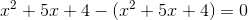Quantity B':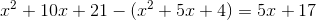Now, it's easy to see that for different values ofQuantity B may be greater, lesser, or equal to Quantity A.

Sinceis not restricted in its possible values, the relationship cannot be determined.

### Example Question #603 : Arithmetic

Rhoda can prunerhododendrons inhours, while Rhonda can pruneinhours. If a work day ishours, how many rhododendrons will Rita have to prune per hour so that the team prunes the garden'srhododendrons?Explanation:

To find out how many rhododendrons Rita will need to prune in an hour, we must first find out how many she needs to prune.

If Rhoda can prunerhododendrons inhours, then she can pruneinhours, and if Rhonda can pruneinhours, she can pruneinhours.

If this is not readily apparent, it can be found by finding out how many each prunes in one hour, then multiplying by.

Rhoda: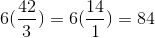Rhonda: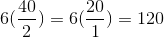Between Rhoda and Rhonda,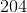of therhododendrons can be pruned, leavingfor Rita.

Since she hashours for the task, her rate of pruning can be found to be: